# 不同相变材料蓄热特性及液相分数无量纲准则方程式拟合The Heat Storage Characteristics of Different Phase Change Materials and the Liquid Phase Fraction Dimensionless Criterion Equation Fitting

DOI: 10.12677/MOS.2021.101014, PDF, HTML, XML, 下载: 49  浏览: 101  国家自然科学基金支持

Abstract: ，拟合得到液相分数的SteF0Sc1/4Ra1/6相关函数。 The main application of numerical simulation method is to study the three-tube phase change heat accumulator, which provides a reference for filling the heat accumulator with three different phase change materials (RT82, RT50, LA). Dimensionless parameters and liquid phase fitting fractions are obtained by fitting. Among them, low melting point RT50 and LA average Nusselt number have a rising stage. SteF0Ra1/6 for different phase change materials and different heat storage methods during the melting process are obtained. Correlation function is verified by the fitting function and obtained by different Stephen number pairs, the results show that the liquid fraction function of the model has high applicability in the range of 0.0765~0.6060 of Ste; the initial temperature below the melting point is introduced. The temperature parameter Sc1/4 is fitted to obtain the SteF0Sc1/4Ra1/6 correlation function of the liquid fraction.

1. 引言

2. 物理模型和数学模型

2.1. 物理模型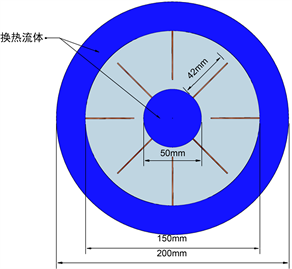Figure 1. Model 1 structure diagram

2.2. 数学模型

${G}_{r}=\frac{g\beta \left({T}_{w}-{T}_{m}\right){D}^{3}}{{v}^{2}}$ (1)

${R}_{a}={P}_{r}\cdot {G}_{r}$ (2)

$\stackrel{¯}{Nu}=\frac{\stackrel{¯}{h}\cdot D}{k}$ (3)

2.3. 数值计算方法和模型考核

2.3.1. 数值计算方法

2.3.2. 模拟方法验证Figure 2. Comparison of numerical simulation results and literature data

2.3.3. 物性条件

1) 相变材料相变过程中采用层流模型，为低速非稳态不可压流动过程；

2) 相变材料和管壁材料为均质和各向同性；

3) 不考虑粘性耗散，密度采用Boussinesq假设，物性参数如表1Table 1. Physical property parameter table

3. 不同相变材料的蓄热特性

3.1. RT82、RT50、LA相变材料的蓄热特性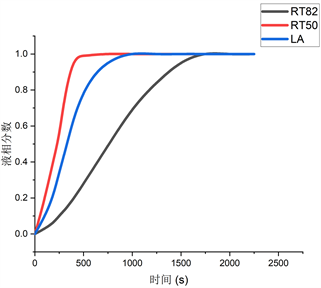(a) 液相分数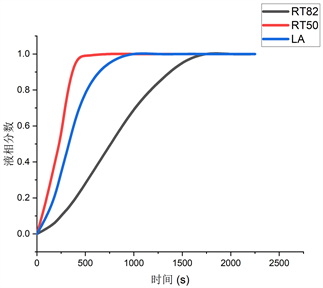(b) 平均温度

Figure 3. RT82, RT50, LA melting process

3.2. 液相对流对蓄热特性的影响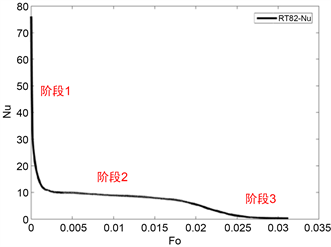(a) RT82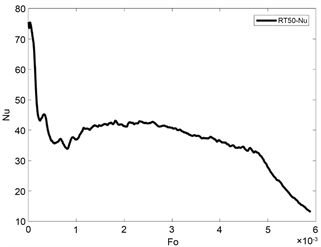(b) RT50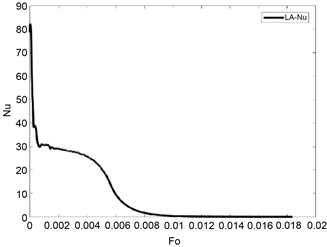(c) LA

Figure 4. $\stackrel{¯}{Nu}$ change of Phase change material heat storage process graph

4. 液相分数的无量纲准则方程式拟合

4.1. 不同相变材料液相分数拟合

${S}_{te}=\frac{{C}_{p}\left({T}_{w}-{T}_{m}\right)}{L}$ (4)

Cpl为液态比热容，L为相变材料的相变潜热值，Tm为PCM的相变温度，Tw为加热表面温度。PCM液相分是函数f与无量纲参数Ste、Fo、Ra相关，其中定义变量x为 ${S}_{te}{F}_{o}{R}_{a}^{\frac{1}{6}}$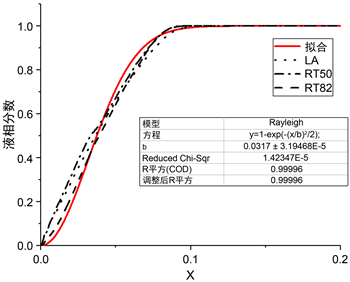Figure 5. x Fitting with liquid fraction

$f=1-{\text{e}}^{\frac{-{\left(\frac{X}{0.0217}\right)}^{2}}{2}}$ (5)

4.2. 不同蓄热方式液相分数拟合

1) 只有内部加热： ${T}_{w}={T}_{in}=363.15\text{\hspace{0.17em}}\text{K}$$R={R}_{m}$$q=0$

2) 只有外部加热： ${T}_{w}={T}_{out}=363.15\text{\hspace{0.17em}}\text{K}$$R={R}_{m}$$q=0$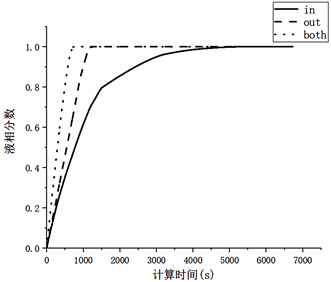Figure 6. Liquid fraction of different heat storage methods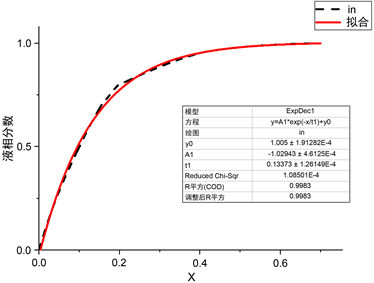Figure 7. Fitting results of liquid phase fraction of the internal heat storage method

$f=1-1.03{\text{e}}^{\frac{-x}{0.134}}$ (6)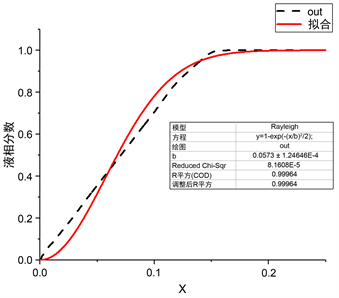Figure 8. Fitting results of liquid fraction of external wall heat storage method

$f=1-{\text{e}}^{\frac{-{\left(\frac{x}{0.0573}\right)}^{2}}{2}}$ (7)

4.3. 不同斯蒂芬数对拟合函数的影响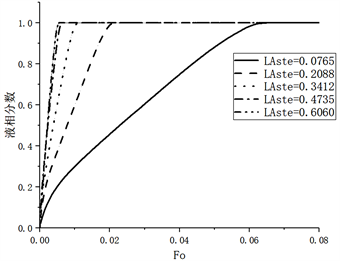Figure 9. Variation of liquid fraction fractions with different Stephen numbers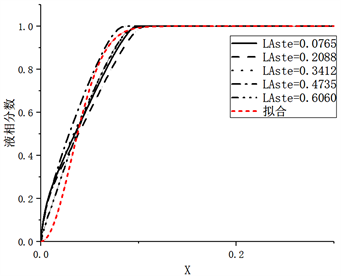Figure 10. Liquid phase fraction fitting with different Stephen numbers

4.4. 不同初温参数对拟合函数的影响

${S}_{c}=\frac{{C}_{p}\left({T}_{m}-{T}_{init}\right)}{L}$ (8)

Cp为比热容，L为相变材料的相变潜热值，Tm为PCM的相变温度，Tinit为初始温度。

$f=1-{\text{e}}^{\frac{-{\left(\frac{x}{0.04756}\right)}^{2}}{2}}$ (9)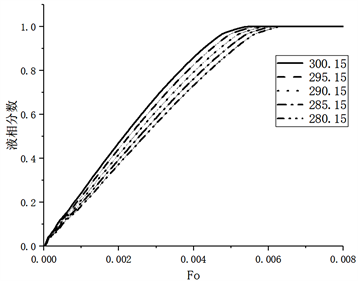Figure 11. Liquid fraction of LA materials at different initial temperatures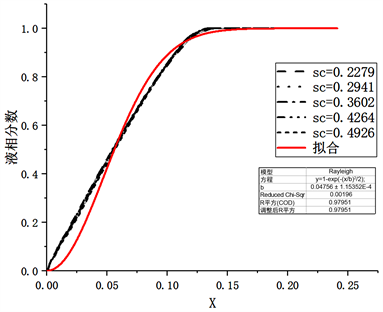Figure 12. Fitting results of different Sc parameters

5. 结论

1) 不同熔点的相变材料虽然自然对流强度变化存在较大差异，但存在相似的熔化阶段。

2) 对不同相变材料、内外管不同蓄热方式、不同蓄热温度拟合得到不同相变材料熔化过程的液相分数相关函数(自变量x为 ${S}_{te}{F}_{o}{R}_{a}^{\frac{1}{6}}$ )，函数准确度较好，可以预测在该模型内相变材料的液相分数变化。

3) 考虑低于熔点的初温参数对蓄热器模型的影响，通过引入初温参数 ${S}_{c}^{-\frac{1}{4}}$，进过拟合得到液相分数与 ${S}_{te}{F}_{o}{S}_{c}^{-\frac{1}{4}}{R}_{a}^{\frac{1}{6}}$ 相关函数，可用于预测。

  Agyenim, F., Eames, P. and Smyth, M. (2009) A Comparison of Heat Transfer Enhancement in a Medium Temperature Thermal Energy Storage Heat Exchanger Using Fins. Solar Energy, 83, 1509-1520. https://doi.org/10.1016/j.solener.2009.04.007  杨莺, 梁艳南, 周孑民, 等. 壳管式相变蓄热器传热效率研究[J]. 热科学与技术, 2011(3): 226-230.  李永辉, 马素霞, 谢豪. 管翅式相变蓄热器性能的实验研究[J]. 可再生能源, 2014(5): 574-578.  Yuan, Y., Cao, X., Xiang, B., et al. (2016) Effect of Installation Angle of Fins on Melting Characteristics of Annular Unit for Latent Heat Thermal Energy Storage. Solar Energy, 136, 365-378. https://doi.org/10.1016/j.solener.2016.07.014  Vogel, J., Keller, M. and Johnson, M. (2020) Numerical Mod-eling of Large-Scale Finned Tube Latent Thermal Energy Storage Systems. Journal of Energy Storage, 29, Article ID: 101389. https://doi.org/10.1016/j.est.2020.101389  Kukreja, N., Gupta, S.K. and Rawat, M. (2020) Perfor-mance Analysis of Phase Change Material Using Energy Storage Device. Materials Today: Proceedings, 26, 913-917. https://doi.org/10.1016/j.matpr.2020.01.139  Abdulateef, J., Mahdi, M.S. and Hasan, A.F. (2020) Experimental Evaluation of Thermal Performance of Two Different Finned Latent Heat Storage Systems. Case Studies in Thermal Engineering, 21, Article ID: 100675.  Cao, X.L., Zhang, N., Yuan, Y.P. and Luo, X.L. (2020) Thermal Performance of Triplex-Tube Latent He Storage Exchanger: Simultaneous Heat Storage and Hot Water Supply via Condensation Heat Recovery. Renewable Energy, 157, 616-625. https://doi.org/10.1016/j.renene.2020.05.059  赵明, 田扬, 胡明禹, 张峰鸣. 三套管式相变蓄热器分形肋片设计及(火积)耗散分析[J]. 热能动力工程, 2020, 35(2): 148-154.  高丽媛, 杨宾, 郝梦琳, 刘杰梅. 不同石墨填料对相变材料性能的影响[J]. 现代化工, 2019, 39(4): 91-94.  赵思勰, 晏华, 李云涛, 汪宏涛, 阚宸玺. 膨胀石墨基定型相变材料的性能研究[J]. 当代化工, 2017, 46(10): 2038-2041.  李云涛, 晏华, 汪宏涛, 余荣升. 膨胀石墨基复合相变材料的结构与性能研究[J]. 材料研究学报, 2016, 30(7): 545-552.  蒋自鹏, 铁生年. 膨胀石墨/芒硝复合定形相变材料制备及性能研究[J]. 材料导报, 2016, 30(12): 55-60.  Liu, M., Sun, Y.P. and Bruno, F. (2020) A Review of Numerical Modelling of High-Temperature Phase Change Material Composites for Solar Thermal Energy Storage. Journal of Energy Storage, 29, Article ID: 101378. https://doi.org/10.1016/j.est.2020.101378  王学晨, 张兴祥, 吴世臻, 牛建津. 相变材料及相变材料微胶囊/聚乙烯共混物的结构与性能[J]. 高分子材料科学与工程, 2005(4): 149-152.  Al-Abidi, A.A., Mat, S., Sopian, K., et al. (2013) Experimental Study of PCM Melting in Triplex Tube Thermal Energy Storage for Liquid Desiccant Air Conditioning System. Energy and Buildings, 60, 270-279. https://doi.org/10.1016/j.enbuild.2013.01.031